International
Tables for
Crystallography
Volume B
Reciprocal space
Edited by U. Shmueli

International Tables for Crystallography (2006). Vol. B. ch. 4.5, pp. 477-478   | 1 | 2 |

## Section 4.5.2.6.5. Difference Fourier synthesis

R. P. Millanea*

#### 4.5.2.6.5. Difference Fourier synthesis

| top | pdf |

Difference Fourier syntheses are widely used in both protein and small-molecule crystallography to detect structural errors or to complete partial structures (Drenth, 1994). The difficulty in applying difference Fourier techniques in fibre diffraction is that the individual observed amplitudes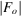are not available. However, difference syntheses have found wide use in fibre diffraction analysis, one of the earliest applications being to polycrystalline fibres of polynucleotides (e.g. Arnott et al., 1967). Calculation of a three-dimensional difference map (for the unit cell) from Bragg fibre diffraction data requires that the observed intensity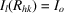be apportioned among the contributing intensities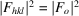. There are two ways of doing this. The intensities may be divided equally among the contributing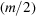reflections [i.e.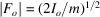], or they may be divided in the same proportions as those in the model, i.e.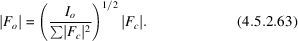The advantage of the former is that it is unbiased, and the advantage of the latter is that it may be more accurate but is biased towards the model. Equal division of the intensities is often (but not always) used to minimize model bias. Once the observed amplitudes have been apportioned, an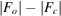map can be calculated as in conventional crystallography, although noise levels will be higher owing to errors in apportioning the amplitudes. As a result of overlapping of the reflections, a synthesis based on coefficients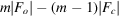gives a more accurate estimate of the true density than does one based on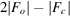, as is described below. Difference syntheses for polycrystalline specimens calculated in this way have been used, for example, to locate cations and water molecules in polynucleotide and polysaccharide structures (e.g. Cael et al., 1978), to help position molecules in the unit cell (e.g. Chandrasekaran et al., 1994) and to help position side chains, and have also been applied in neutron fibre diffraction studies of polynucleotides (Forsyth et al., 1989).

Sim (1960)has shown that the mean-squared error in difference syntheses can be minimized by weighting the coefficients based on the agreement between the calculated and observed structure amplitudes. Such an analysis has recently been conducted for fibre diffraction, and shows that the optimum difference synthesis is obtained by using coefficients (Millane & Baskaran, 1997; Baskaran & Millane, 1999a)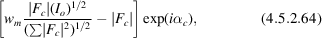where m is the number of degrees of freedom as defined in Section 4.5.2.6.1. If the reflections contributing to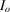are either all centric or all acentric, then the weights are given by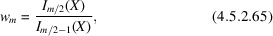where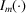denotes the modified Bessel function of the first kind of order m, and X is given by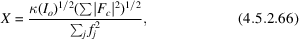where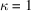for centric reflections and 2 for acentric reflections. The form of the weighting function is more complicated if both centric and acentric reflections contribute, but it can be approximated as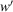given by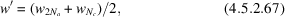where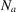andare the number of acentric and centric reflections, respectively, contributing. Use of the weighted maps reduces bias towards the model (Baskaran & Millane, 1999b).

For continuous diffraction data from noncrystalline specimens, the situation is essentially identical except that one works in cylindrical coordinates. Referring to equations (4.5.2.7)and (4.5.2.10), the desired difference synthesis,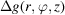, is the Fourier–Bessel transform of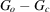where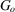anddenote the observed and calculated, respectively, Fourier–Bessel structure factors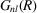. Sinceis not known, the synthesis is based on the Fourier–Bessel transform of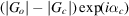, where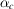is the phase of. As in the polycrystalline case, the individual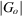need to be estimated from the data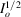given by equation (4.5.2.17), and can be based on either equal division of the data, or division in the same proportion as the amplitudes from the model.

Namba & Stubbs (1987a)have shown that the peak heights in a difference synthesis are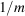times their true value, as opposed to half their true value in a conventional difference synthesis. The best estimate of the true map is therefore provided by a synthesis based on the coefficients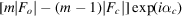, rather than on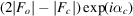. Test examples showed that the noise in the synthesis can be reduced by using a value for m that is fixed over the diffraction pattern and approximately equal to the average value of m over the pattern (Namba & Stubbs, 1987a). Difference Fourier maps for noncrystalline systems have been used in studies of helical viruses to locate heavy atoms, to correct errors in atomic models and to locate water molecules (Mandelkow et al., 1981; Lobert et al., 1987; Namba, Pattanayek & Stubbs, 1989; Wang & Stubbs, 1994).

### ReferencesArnott, S., Wilkins, M. H. F., Fuller, W. & Langridge, R. (1967). Molecular and crystal structures of double-helical RNA III. An 11-fold molecular model and comparison of the agreement between the observed and calculated three-dimensional diffraction data for 10- and 11-fold models. J. Mol. Biol. 27, 535–548.Google ScholarBaskaran, S. & Millane, R. P. (1999a). Bayesian image reconstruction from partial image and aliased spectral intensity data. IEEE Trans. Image Process. 8, 1420–1434.Google ScholarBaskaran, S. & Millane, R. P. (1999b). Model bias in Bayesian image reconstruction from X-ray fiber diffraction data. J. Opt. Soc. Am. A, 16, 236–245.Google ScholarCael, J. J., Winter, W. T. & Arnott, S. (1978). Calcium chondroitin 4-sulfate: molecular conformation and organization of polysaccharide chains in a proteoglycan. J. Mol. Biol. 125, 21–42.Google ScholarChandrasekaran, R., Radha, A. & Lee, E. J. (1994). Structural roles of calcium ions and side chains in welan: an X-ray study. Carbohydr. Res. 252, 183–207.Google ScholarDrenth, J. (1994). Principles of protein X-ray crystallography. New York: Springer-Verlag.Google ScholarForsyth, V. T., Mahendrasingam, A., Pigram, W. J., Greenall, R. J., Bellamy, K., Fuller, W. & Mason, S. A. (1989). Neutron fibre diffraction study of DNA hydration. Int. J. Biol. Macromol. 11, 236–240.Google ScholarLobert, S., Heil, P. D., Namba, K. & Stubbs, G. (1987). Preliminary X-ray fibre diffraction studies of cucumber green mottle mosaic virus, watermelon strain. J. Mol. Biol. 196, 935–938.Google ScholarMandelkow, E., Stubbs, G. & Warren, S. (1981). Structures of the helical aggregates of tobacco mosaic virus protein. J. Mol. Biol. 152, 375–386.Google ScholarMillane, R. P. & Baskaran, S. (1997). Optimal difference Fourier synthesis in fibre diffraction. Fiber Diffr. Rev. 6, 14–18.Google ScholarNamba, K., Pattanayek, R. & Stubbs, G. J. (1989). Visualization of protein–nucleic acid interactions in a virus. Refined structure of intact tobacco mosaic virus at 2.9 Å resolution by X-ray fibre diffraction. J. Mol. Biol. 208, 307–325.Google ScholarNamba, K. & Stubbs, G. (1987a). Difference Fourier syntheses in fibre diffraction. Acta Cryst. A43, 533–539.Google ScholarSim, G. A. (1960). A note on the heavy atom method. Acta Cryst. 13, 511–512.Google ScholarWang, H. & Stubbs, G. J. (1994). Structure determination of cucumber green mottle mosaic virus by X-ray fibre diffraction. Significance for the evolution of tobamoviruses. J. Mol. Biol. 239, 371–384.Google Scholar